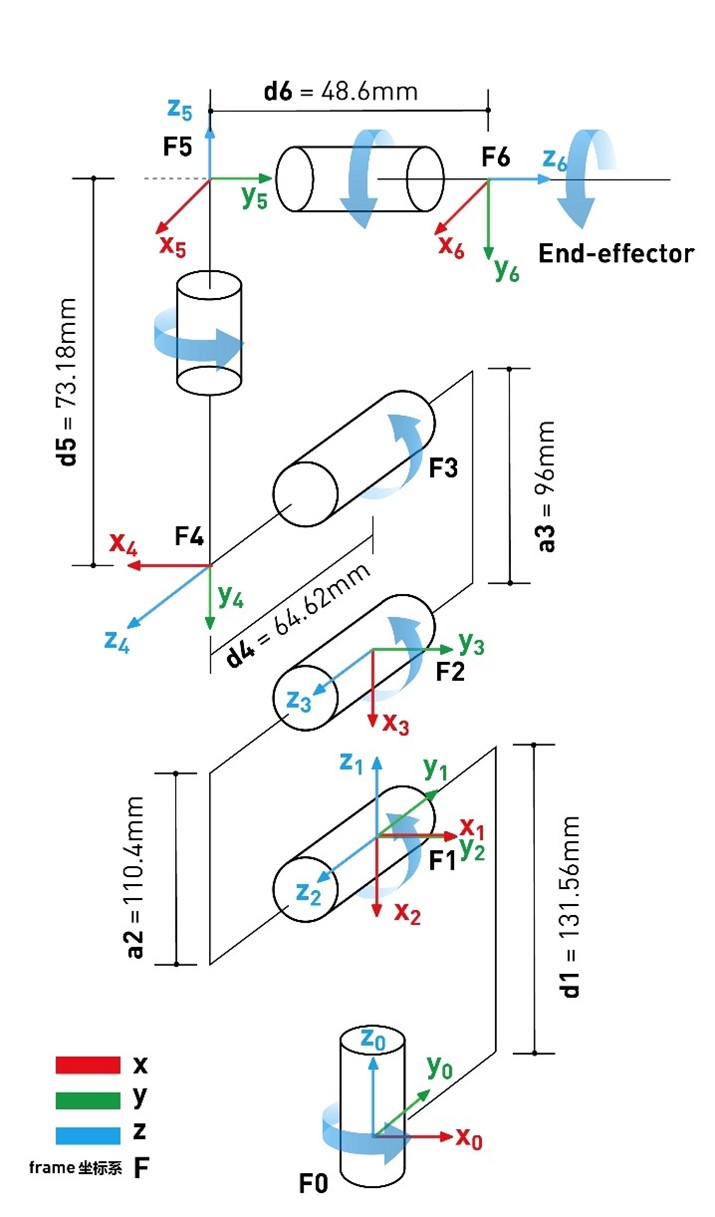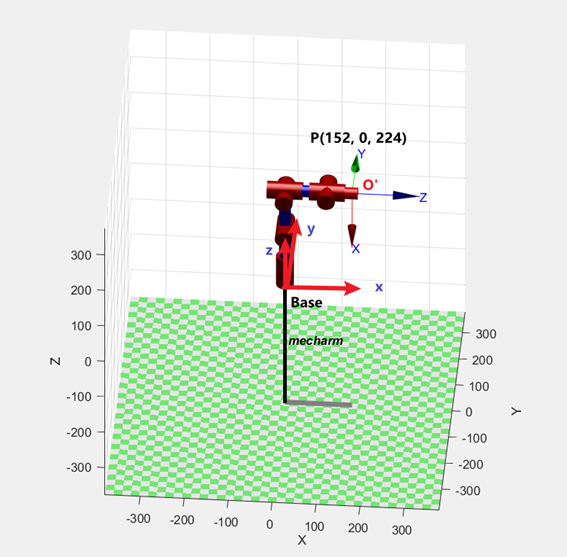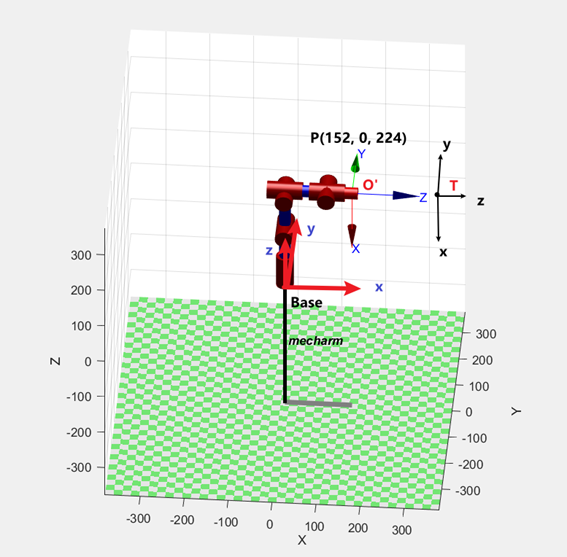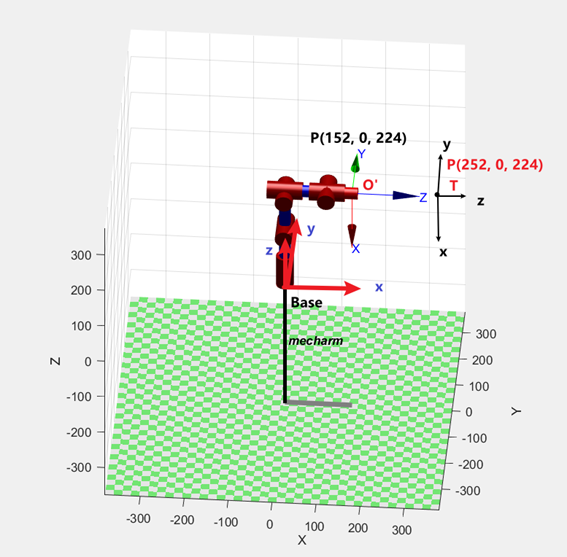# myCobot 280 algorithm

## 1 Structural Parameters

### 1.1 DH Parameters of Robotic Arm

joint theta d a alpha offset
1 q1 131.22 0 1.5708 0
2 q2 0 -110.4 0 -1.5708
3 q3 0 -96 0 0
4 q4 63.4 0 1.5708 -1.5708
5 q5 75.05 0 -1.5708 1.5708
6 q6 45.6 0 0 0

### 1.2 Kinematic Model## 2 Coordinate System Introduction

### 2.1 Tool Coordinate SystemThe figure shows the robot model of Mecharm270. Base in the figure represents the base coordinate system of the robot, O' represents the end flange coordinate system, and point P represents the position of the end of the manipulator relative to the base coordinate system (x=152, y=0 , z=224)

Extend a certain pose on the basis of the end flange, and regard the set tool point as the end of the machine:T in the figure is the set tool coordinate system. The posture of this coordinate system is consistent with O’, and the relative displacement of the origin has occurred. Use the python function to set the tool coordinate system:

• set_tool_reference([x, y, z, rx, ry, rz]) //Set tool coordinate system
• set_end_type(1) //Set the end coordinate system type as tool
• Assume that the tool coordinate system T is not rotated relative to O' (rx = ry = rz = 0)
• Assume that the origin of the tool coordinate system T is in the coordinate system O’ at (x = 0, y = 0, z = 100mm)
• The final tool coordinate system parameter is set_tool_reference(0, 0, 100, 0, 0, 0)Since the tool coordinate system is set, the end of the robot extends from O' to T at this time, and the coordinates of the end of the machine read at this time become (152+100, 0, 224), and the coordinate posture movement will revolve around the tool point T rotate.

### 2.2 World Coordinate SystemSection 2 introduces that by setting the tool coordinate system, the end coordinate system of the manipulator can be extended to a certain pose. We can also extend a certain pose on the basis of the base coordinate system of the manipulator by setting the world coordinate system. The set world coordinate system will replace the original Base coordinate system and become the new base coordinate system.

W in the figure is the set world coordinate system. The posture of this coordinate system is consistent with Base, and the relative displacement of the origin has occurred. Use the python function to set the world coordinate system:

• set_world_reference([x, y, z, rx, ry, rz]) //Set the world coordinate system
• set_reference_frame(1) //Set the base coordinate system type to the world
• Assuming that the world coordinate system W has not rotated relative to Base(rx = ry = rz = 0)
• Suppose the origin of the world coordinate system W is in the coordinate system Base (x = 0, y = 0, z = -100mm)
• The final world coordinate system parameter is set_world_reference(0, 0, -100, 0, 0, 0)

Since the world coordinate system is set, the origin of the robot extends from Base to W at this time, and the O’ coordinate read at this time becomes (152, 0, 224+100).